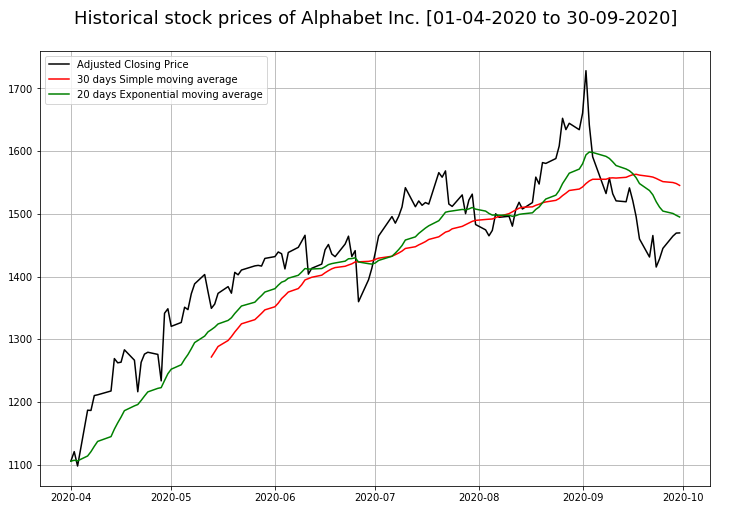﻿ Pandas: Create a plot of adjusted closing prices, thirty days simple moving average and exponential moving average - w3resource# Pandas: Create a plot of adjusted closing prices, thirty days simple moving average and exponential moving average

## Pandas: Plotting Exercise-15 with Solution

Write a Pandas program to create a plot of adjusted closing prices, 30 days simple moving average and exponential moving average of Alphabet Inc. between two specific dates.

Use the alphabet_stock_data.csv file to extract data.

What is Simple Moving Average (SMA)?
In financial applications a simple moving average (SMA) is the unweighted mean of the previous n data. However, in science and engineering, the mean is normally taken from an equal number of data on either side of a central value. This ensures that variations in the mean are aligned with the variations in the data rather than being shifted in time.

What Is an Exponential Moving Average (EMA)?
From investopedia.com - An exponential moving average (EMA) is a type of moving average (MA) that places a greater weight and significance on the most recent data points. The exponential moving average is also referred to as the exponentially weighted moving average. An exponentially weighted moving average reacts more significantly to recent price changes than a simple moving average (SMA), which applies an equal weight to all observations in the period.alphabet_stock_data:

alphabet_stock_data Table

Date Open High Low Close Adj Close Volume
2020-04-01 1122 1129.689941 1097.449951 1105.619995 1105.619995 2343100
2020-04-02 1098.26001 1126.859985 1096.400024 1120.839966 1120.839966 1964900
2020-04-03 1119.015015 1123.540039 1079.810059 1097.880005 1097.880005 2313400
2020-04-06 1138 1194.660034 1130.939941 1186.920044 1186.920044 2664700
... ... ... ... ... ... ...
... ... ... ... ... ... ...
2020-09-29 1470.390015 1476.662964 1458.805054 1469.329956 1469.329956 978200
2020-09-30 1466.800049 1489.75 1459.880005 1469.599976 1469.599976 1700600

Sample Solution:

Python Code :

``````import pandas as pd
import matplotlib.pyplot as plt
start_date = pd.to_datetime('2020-4-1')
end_date = pd.to_datetime('2020-9-30')
df['Date'] = pd.to_datetime(df['Date'])
new_df = (df['Date']>= start_date) & (df['Date']<= end_date)
df1 = df.loc[new_df]
stock_data = df1.set_index('Date')
stock_data['SMA_30_days'] = stock_data.iloc[:,4].rolling(window=30).mean()
plt.figure(figsize=[15,10])
plt.grid(True)
plt.title('Historical stock prices of Alphabet Inc. [01-04-2020 to 30-09-2020]\n',fontsize=18, color='black')
plt.plot(stock_data['SMA_30_days'],label='30 days Simple moving average', color='red')
plt.plot(stock_data['EMA_20_days'],label='20 days Exponential moving average', color='green')
plt.legend(loc=2)
plt.show()
``````

Sample Output:

``````

Python Code Editor:

Have another way to solve this solution? Contribute your code (and comments) through Disqus.

What is the difficulty level of this exercise?

Test your Python skills with w3resource's quiz

﻿

## Python: Tips of the Day

Trenary operator:

```>>> "Python ROCK" if True else " I AM GRUMPY"
"Python ROCK"
```